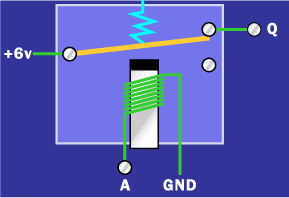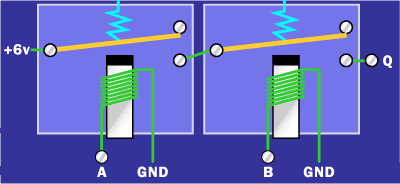## Audio

[Audio][slideshow]

To know the basics of Logic Gates and their applications, click on the links below.

TAKE A LOOK : LOGIC GATES

TAKE A LOOK : FLIP FLOPS

With the invention of Logic gates, we can design electronic circuits that can simply work as a calculator to high end devices that can be even used for scientific purposes. Earlier only humans could add and subtract numbers. But with the applications of Boolean gates like Adders, Subtractors, Counters and so on it is possible to create almost any logic.

With this post, we are going to help you and thus understand better, the logic behind using gates. The steps are simple and creative. But for the implementation requires a relay and a 6 volt battery. The very first implementation of Boolean gates also included relays. But the technology has gone far beyond that they have been replaced by sub-microscopic transistors etched onto silicon chips. Relays consume some amount of power, and they are bigger in size. But the transistors used these days are microscopic and are faster than relays. They also consume very less power when compared to a relay.

But you will get a clear idea about the working by using relays. They are also practically very simple to use. All you need to know is know the basics of gates and their truth table. Enter the input values and take the output values.

To know more about setting up a relay, and it’s working take a look at this link.

TAKE A LOOK : WORKING OF RELAYS

As we know there are only two states for a Gate – a HIGH state and a LOW state. In a relay, these states will be represented by two voltages. The HIGH state is represented by 6 volts and the LOW state to be 0 volts. A 6 volt battery is required to power up the circuit.

## NOT Gate

Take a look at the picture below to get a clear idea on how a NOT Gate is implemented. From the circuit it is clear that when you apply 6 volts to A, you get 0 volts in Q and when you apply 0 volts to A you get 6 volts in Q.NOT GATE Implementation

## AND Gate

As an AND bit requires a minimum of 2 inputs, two relays will be needed. The figure is shown below.AND GATE Implementation

From the figure it is clear that if 6 volts it applied to both A and B, you get the output Q=6 volts. For any other combination of A and B, the output will be 0 volts. The same circuit can be changed into an OR Gate by hooking two wires for A and B together. You can also use two relays in parallel.

You can also make the circuit fancier by reading the outputs on bulbs. That is, when a HIGH state occurs, LIGHT = ON. When a LOW state occurs, LIGHT = OFF.

Thus the three basic Gates NOT, AND and OR can be implemented easily.

With the help of the basics above you can easily implement 8-bit ripple carry adders and so on. All these basics are also the basics for the working of computers.

[Lights][grids]